Most Affordable JEE | NEET | 8,9,10 Preparation by Kota's Top IITian Doctor Faculties

# Rotational Motion Problems and Solutions Physics Class 11 Important Question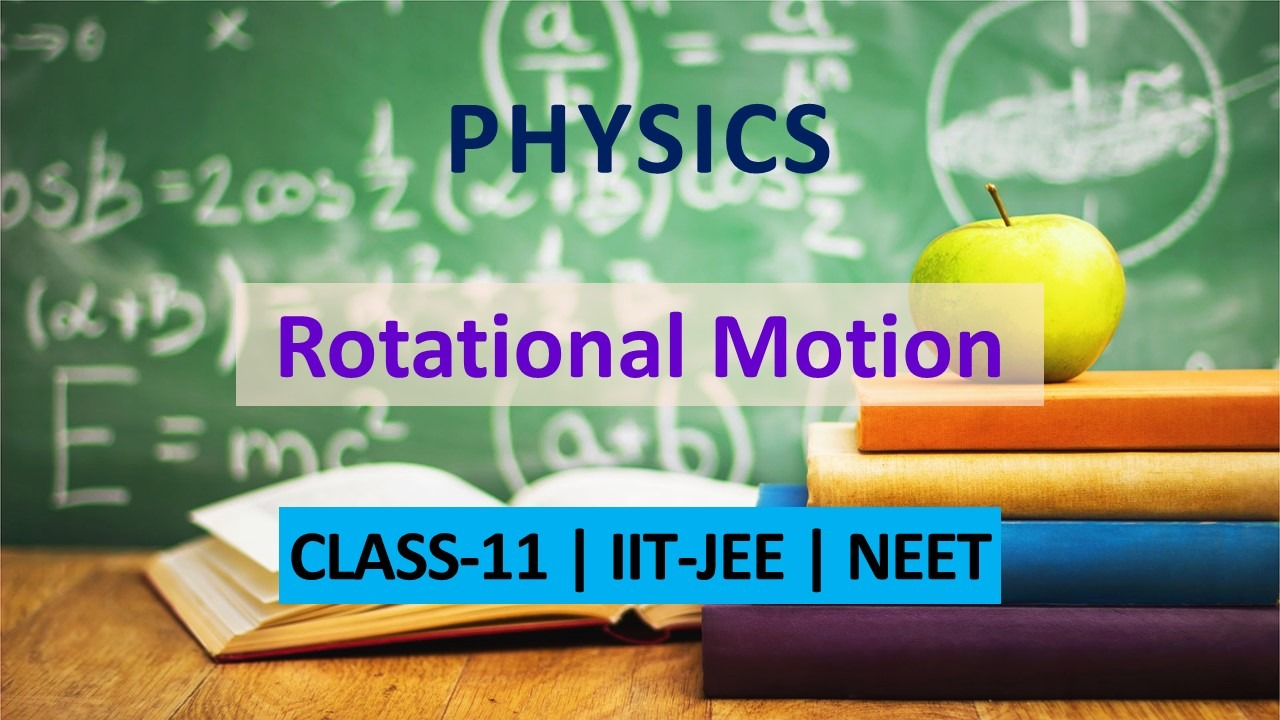Get Rotational Motion important problems with solutions for Board exams. View 11th Physics important questions developed by top IITian faculties for exam point of view. These important problems with solutions will play significant role in clearing concepts related to rotational motion chapter. This question bank is designed by keeping NCERT in mind and the questions are updated with respect to upcoming Board exams. You will get here all the important questions for class 11 & 12 Physics chapters.In this article you will learn the concept of rotational motion with important problems and having their solutions too.   Click Here for Detailed Notes of system of particles and rotational motion along with notes of any chapter and other subjects.
Q. Which component of linear momentum does not contribute to angular momentum ?
Ans. Radial component of linear momentum.
Q. What is the cause and outcome in a rotational motion ?
Ans. Cause of rotational motion – Torque Out come in rotational motion – Angular momentum
Q. Is it possible to open a pen up with one finger ? Why ?
Ans. Is it possible to open a pen up with one finger ? Why ?
Q. A rifle barrel has a spiral groove which imparts spin to the bullet. Why ?
Ans. Angular momentum gained by the bullet provides better accuracy.
Q. For uniform circular motion, does the direction of the centripetal force depend on the sense of rotation (i.e., clock wise or anti clockwise) ?
Ans. No, direction of centripetal force remains constant either it is clockwise and anticlockwise rotation.
Q. If a body is rotating, is it necessarily being acted upon by an external torque ?
Ans. No, torque is required only for producing angular acceleration. For uniform rotation, no torque is needed.
Q. A projectile acquires angular momentum about the point of projection during flight. Does it violate the conservation of angular momentum ?
Ans. A projectile acquires angular momentum about the point of projection during flight. Does it violate the conservation of angular momentum ?
Q. Give the physical significance of moment of inertia ?
Ans. The moment of inertia plays the same role in rotational motion as the mass does in linear motion.
Q. The moment of inertia plays the same role in rotational motion as the mass does in linear motion.
Ans. Yes, moment of inertia of a body changes with the change of the axis of rotation.
Q. Is there any difference between moment of inertia and rotational inertia ? or Why is moment of inertia called rotational inertia ?
Ans. The moment of inertia is another name for rotational inertia. The moment of inertia is called rotational interia for the reason that it gives the measure of inertia in rotational motion.
Q. Why are the spokes fitted in a cycle wheel ?
Ans. Why are the spokes fitted in a cycle wheel ?
Q. Two solid spheres of same mass are made of metals of different densities. Which of them has a larger moment of inertia about a diameter ?
Ans. Two solid spheres of same mass are made of metals of different densities. Which of them has a larger moment of inertia about a diameter ?
Q. A ring and a circular disc of different material have equal masses and equal radii. Which one will have a larger moment of inertia about an axis passing through its centre of mass and perpendicular to its plane ?
Ans. A ring has a larger moment of inertia because its entire mass is at a larger distance from the axis of rotation than the average distance of the mass particles of the disc.
Q. A disc of metal is melted and recast in the form of a solid sphere. What will happen to the moment of inertia about a vertical axis passing through the centre ?
Ans. The moment of inertia will decrease, because the material particles will, on the average come closer to the axis.
Q. Is friction necessary for a body to roll ? Why ?
Ans. If no other external force acts on a body, friction is necessary for a body to roll. Frictional force being tangential provides torque. Any force at the centre of mass will provide no torque and so cannot roll the body.
Short Answer Type [2 & 3 Marks]
Q. Can a body in translatory motion have angular momentum ? Explain.
Ans. Yes, a body in translatory motion shall have angular momentum unless the fixed point about which angular momentum is taken lies on the line of motion of the body. This follows from $|L|=r p \sin \phi \cdot L=0,$ only when $\phi=0$ or $\phi=180^{\circ}$
Q. A planet revolves around a massive star in a highly elliptical orbit. Is its angular momentum constant over the entire orbit ?
Ans. Yes, angular momentum of the planet is constant over the entire orbit. This is because revolution of planet around the star is under the effect of gravitational force between the star and the planet. This is a radial force whose torque is zero. Therefore, angular momentum of the planet is constant (vector), whatever be the nature of the orbit.
Q. A star of mass M and radius $10^{6} \mathrm{km}$ rotates about its axis with an angular speed of $10^{-6} \mathrm{s}^{-1}$. What is the angular speed of the star when it collapses (due to inward gravitational forces) to a radius of $10^{4} k m$. Assume that there is no mass loss by the star during collapse.
Ans. Let $I_{1}$ and $\omega_{1}$ be the initial moment of inertia and the angular speed of the star about its own axis of rotation, and $I_{2}$ and $\omega_{2}$ be the corresponding quantities after the star is collapsed. since the collapse is due to the internal forces (gravitational attraction of the outer layers by the inner layers of the star), the angular momentum of the star remains conserved during collapse. Thus,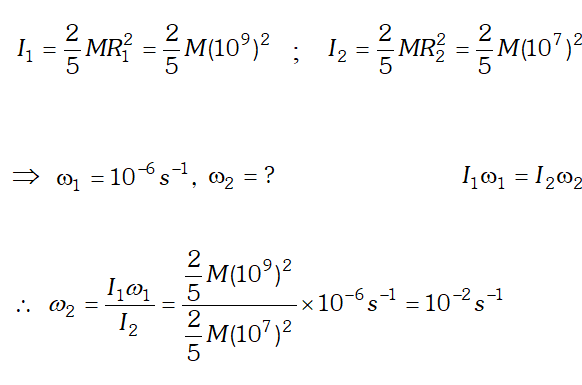The collapsed star rotates with a speed $10^{4}$ times the initial angular speed.
Q. A child stands at the centre of a turn table with his two arms outstretched. The turn table is set rotating with an angular speed of 40 r.p.m. (a) How much is the angular speed of the child, if he folds his hands back reducing the moment of inertia to (2/5) time the initial value ? Assume that the turn table rotates without friction. (b) Show that the childs’s new K.E. of rotation is more than the initial K.E. of rotation. How do you account for this increase in K.E. ? [NCERT]
Ans. Here, initial angular speed, $\omega_{1}=40 \mathrm{rev} / \mathrm{min} ; \omega_{2}=?$ final moment of inertia, $I_{2}=\frac{2}{5} I_{1}$ As no external torque acts in the process, therefore, L= constant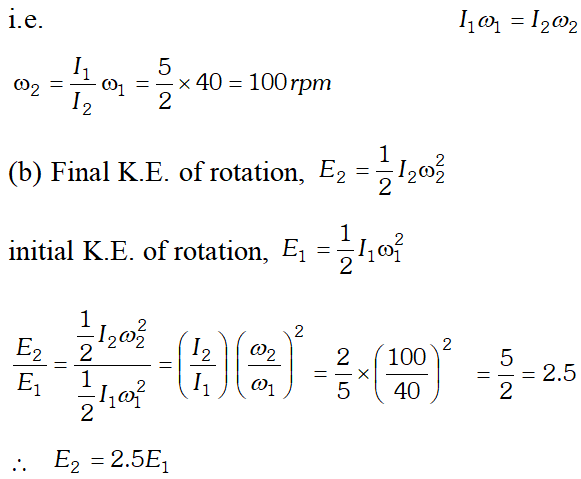i.e. K.E. of rotation increases. This is because the child spends internal energy in folding back his hands.
Q. What is the angular momentum of the projectile when it is at the highest point about an axis through the point of projection ?
Ans. At the highest point of projectile motion the velocity is $u \cos \theta$ acting horizontally. The perpendicular distance between the point of projection and the highest point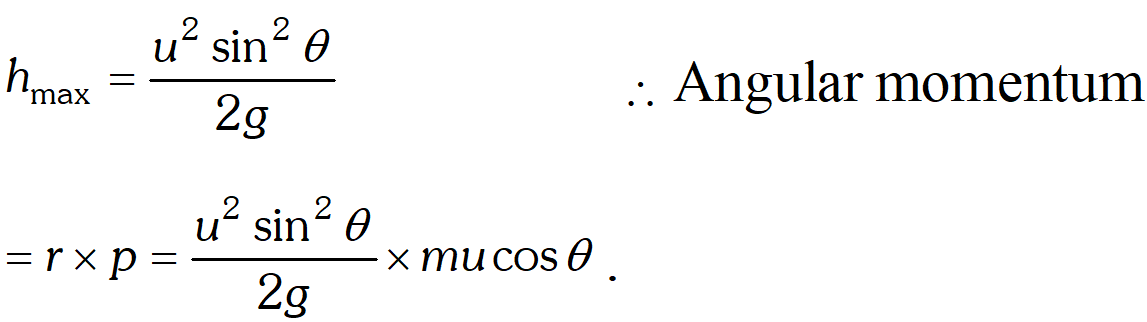Q. A planet moves around the sun under the effect of gravitational force exerted by the sun. Why the torque on the planet due to the gravitational force is zero ?
Ans. The torque on the planet due the sun, $\vec{\tau}=\vec{r} \times \vec{F}$ Where $\vec{r}$ is position vector of the planet $w \cdot t .$ the sun and $\vec{F}$ is the gravitational force on the planet since the gravitational force on the planet acts along the line joining the planet to the sun, the vectors and are always parallel and hence.
Q. A tube of length l is filled with a liquid density . Find the force experienced at one of its edge if rotated about the other edge with an angular velocity .
Ans. Angular velocity will be same for all points. Consider a small portion of length dx at a distance x from the axis. Mass of $d x=\rho . A d x,$ where $A$ is cross sectional area. Force experienced by $d x=m r \omega^{2}=\rho A d x . x \omega^{2}$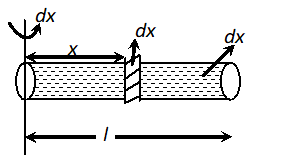Force at the edge $=\int_{0}^{l} \rho A \omega^{2} x d x \Rightarrow F=\rho A \omega^{2} \frac{l^{2}}{2}$
Q. A grindstone has a moment of inertia . A constant torque is applied and the grindstone is found to have a speed of 150 r.p.m. in 10 sec after starting from rest. Calculate the torque.
Ans. Here, Moment of inertia of grindstone, $I=6 \mathrm{kg} m^{2}$ Initial angular velocity, $\omega_{1}=0$ Final angular velocity, $\omega_{2}=2 \pi n=2 \pi \times \frac{150}{60}=5 \pi \mathrm{rad} / \mathrm{sec}$ Time for which torque acts, $t=10 \mathrm{sec} .$ $\therefore$ Angular acceleration $(\alpha)=\frac{\omega_{2}-\omega_{1}}{t}=\frac{5 \pi-0}{10}=\frac{\pi}{2} r a d / \sec ^{2}$ As $\tau=I \alpha \quad \therefore \tau=6 \times \frac{\pi}{2}=3 \pi \mathrm{Ns}$
Q. A disc rotating about its axis with angular speed $\omega_{0}$ is placed lightly (without any transnational pull) on a perfectly frictionless table. The radius of the disc is R. What are the linear velocities of the points A, B and C on the disc shown in fig. ? Will the disc roll in the direction indicated ?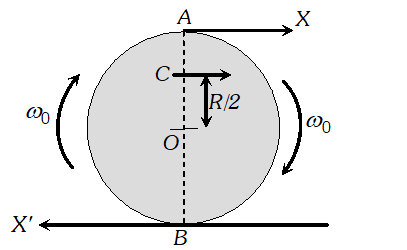[NCERT]
Ans. Using the relation $v=r \omega,$ we get For point $A, v_{A}=R \omega_{0},$ along $A X$ For point $B, v_{B}=R \omega_{0},$ along $B X^{\prime}$ For point $C, v_{C}=\left(\frac{R}{2}\right) \omega_{0}$ parallel to $A X,$ The disc will not rotate, because it is placed on a perfectly frictionless table. Without friction, rolling is not possible.
Q. To maintain a rotor at uniform angular speed of $200 \mathrm{rads}^{-2}$$200 \mathrm{rads}^{-2}$, an engine needs to transmit a torque of 180 N m. What is the power of the engine required ? [NCERT]
Ans. For a ‘uniform’ angular speed of rotation, no torque is needed, provided there is no friction. In practice, however, a torque has to be applied to the rotor to counterbalance the frictional torque. If $\tau$ be the frictional torque, then the required power of the engine transmitting the torque is given by $P=\tau \omega$ when $\omega$ is the angular speed. Here $\tau=180 N m$ and $\omega=200 r a d s^{-1},$ therefore $P=180 N m \times 200 s^{-1}=36000 W=36 k W$
Q. Prove that the torque acting due to a force $\mathrm{F}$ in the $x y$ plane is, $\tau=\mathrm{xF}_{y}-\mathrm{yF}_{\mathrm{x}}$
Ans. We know $\vec{\tau}=\vec{r} \times \vec{F}$. If $\vec{r}=x \hat{i}+y \hat{j}+z \hat{k}$ and $\vec{F}=F_{x} \hat{i}+F_{y} \hat{j}+F_{z} \hat{k},$ we have, $\tau=\tau_{x} \hat{i}+\tau_{y} \hat{j}+\tau_{z} \hat{k}=\left|\begin{array}{ccc}{\hat{i}} & {\hat{j}} & {\hat{k}} \\ {\hat{i}} & {\hat{j}} & {\hat{k}} \\ {x} & {y} & {z} \\ {F_{x}} & {F_{y}} & {F_{z}}\end{array}\right|$ Comparing the coefficients of $\hat{i}, \hat{j}$ and $\hat{k},$ we have $\tau_{z}=x F_{y}-y F_{x}$
Q. Explain why friction is necessary to make the disc roll in the direction indicated. (a) Give the direction of frictional force at B, and the sense of frictional torque, before perfect rolling begins. (b) What is the force of friction after prefect rolling begins ?
Ans. To roll a disc, we require a torque, which can be provided only by a tangential force. As force of friction is the only tangential force in this case, it is necessary. (a) As frictional force at B opposes the velocity of point B, which is to the left, the frictional force must be to the right. The sense of frictional torque will be perpendicular to the plane of the disc and outwards. (b) As frictional force at B decreases the velocity of the point of contact B with the surface, the perfect rollling beings only when velocity of point B becomes zero. Also, force of friction would become zero at this stage.
Q. State the theorems of Parallel axes.
Ans. Parallel AxesTheorem The moment of inertia about an axis passing parallel to the axis through the centre of mass of a rigid body is the sum of the moment of inertia ( $l_{m}$ ) of the body about the axis through centre of mass and the product of its mass $M$ and square of the separation $\left(a^{2}\right)$ between the parallel axis.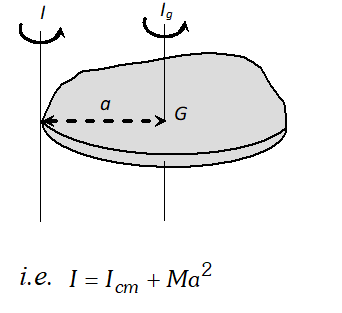Q. State the perpendicular axes theorem.
Ans. Perpendicular Axes Theorem According to this theorem the sum of moment of inertia of a plane lamina about two mutually perpendicular lying in its plane is equal to its moment of inertia about an axis perpendicular axes to the plane of lamina and passing through the point of intersection of first two axes.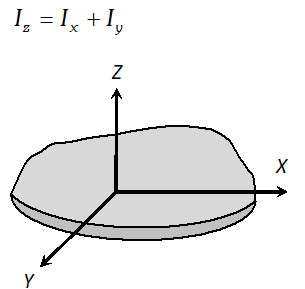Q. In a fly wheel, most of the mass is concentrated at the rim. Explain, why.
Ans. In a fly wheel, most of the mass is concentrated at the rim. Due to this peculiar shape, the flywheel possesses a large value of moment of inertia for the given mass and the radius. If such a wheel gains or loses some rotational energy, it brings about only a very small change in its angular speed. In this way, a flywheel helps to maintain uniform motion.
Q. A rod of length l and mass M held vertically is let go down, without slipping at the point of contact. What is the velocity of the top end at the time of touching the ground ?
Ans. Loss in P.E. = Gain in rotational Kinetic energy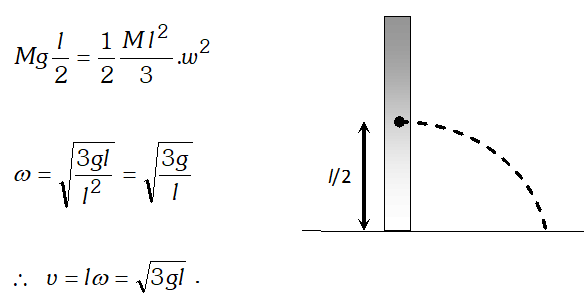Q. Two identical cylinders ‘run a race’ starting from rest at the top of an inclined plane, one slides without rolling and other rolls without slipping. Assuming that no mechanical energy is dissipated in heat, which one will win ?
Ans. When a cylinder slides without rolling, $E=\frac{1}{2} m v_{1}^{2} \Rightarrow v_{1}=\sqrt{\frac{2 E}{m}}$ When the cylinder rolls without slipping $E=\frac{1}{2} m v_{2}^{2}+\frac{1}{2} I w^{2} \Rightarrow E=\frac{1}{2} m v_{2}^{2}+\frac{1}{2}\left(\frac{1}{2} m r^{2}\right) w^{2}$ $=\frac{1}{2} m v_{2}^{2}+\frac{1}{4} m v_{2}^{2}=\frac{3}{4} m v_{2}^{2} \quad[\because v=r w]$ $\therefore v_{2}=\sqrt{\frac{4 E}{3 m}}$ As $v_{1}>v_{2},$ therefore sliding cylinder will win the race,
Q. Why is it more difficult to revolve a stone by tying it to a longer string than by tying it to a shorter string ?
Ans. Consider that a stone of mass $M$ is revolved along a circular path by tying it to a thread of length $l .$ Moment of inertia of stone, $I=M l^{2}$ Suppose that a torque $\tau$ is applied to produce an angular acceleration $\alpha .$ Then, $\tau=I \alpha=\left(M l^{2}\right) \alpha$ Or $\alpha=\frac{\tau}{M l^{2}}$ It follows that to produced the same amount of angular acceleration; the larger torque is required, when longer string is used to revolve a stone of given mass. Hence, it is more difficult to revolve a stone by tying it to a longer string.
Q. Three point mass are located at the vertices of an equilateral triangle of length a. What is the moment of inertia of the system about an axis along the altitude of the triangle passing through ? [NCERT]
Ans. In fig. $A B C$ is an equilateral triangle. Let $A O \perp B C$, so $A O$ is also bisector of $B C$ i.e. $A O$ is median of $\triangle A B C .$ We have to calculate moment of inertia of the system about AO.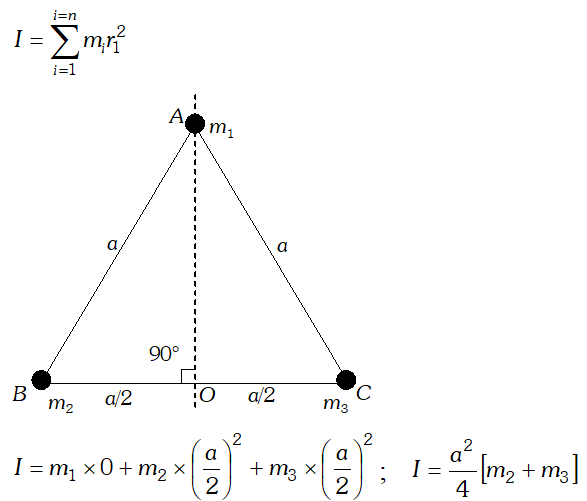Q. A solid cylinder of mass 20 kg rotates about its axis with angular speed $100 \mathrm{s}^{-1}$. The radius of the cylinder is 0.25 m. What is the kinetic energy associated with the rotation of the cylinder ? What is the magnitude of angular momentum of the cylinder about its axis ?
Ans.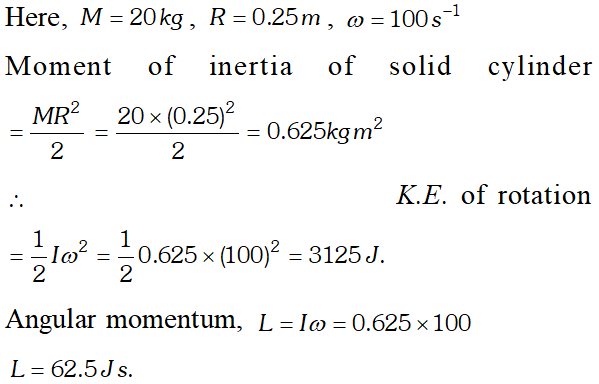Q. What is the moment of inertia of a uniform circular disc of radius R and mass M about an axis passing through its centre and normal to the disc. The moment of inertia of the disc about any of its diameter is given to be $\frac{1}{4} \mathrm{MR}^{2}$. [NCERT]
Ans. We are given, moment of inertia of the disc about any of its diameter $=\frac{1}{4} M R^{2}$ Using theorem of perpendicular axes, moment of inertia of the disc about an axis passing through a point on its edge and normal to the disc $=2 \times \frac{1}{4} M R^{2}=\frac{1}{2} M R^{2}$
Q. A solid cylinder of mass M and radius R is held at rest in a horizontal position. Two strings are wound around the cylinder. As the string gets un-wound, find the tension in the string and acceleration in the cylinder.
Ans. $M g-2 T=M a \quad ; \quad 2 T R=I \alpha=\frac{M R^{2}}{2} \frac{a}{R}$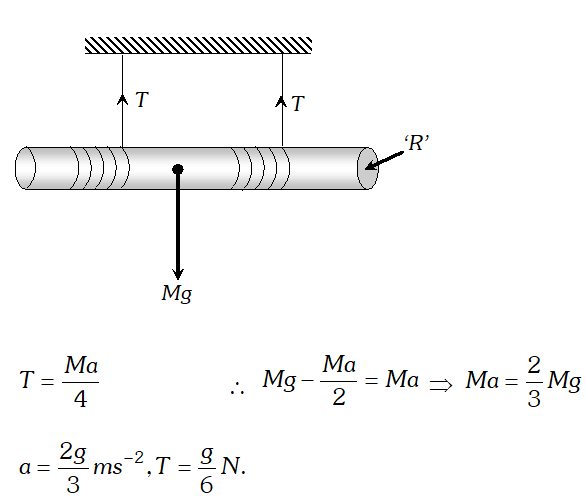Q. Explain, why friction is necessary to make the disc roll in the direction indicated. (a) Give the direction of frictional force at B, and the sense of frictional torque, before perfect rolling begins. (b) What is the force of friction after perfect rolling begins ?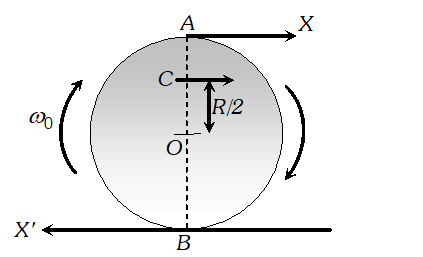Ans. The make the disc roll, a torque is required which in turn requires tangential force on it. Here, in this problem, only frictional force can provide the tangential force. (a) Frictional force at point B opposes the velocity of point B. Therefore, frictional force at the point of contact is in the same direction as the arrow. The diretion of frictional torque is such that it opposes the angular motion. since is normal to the plane of the paper in inward direction, the direction of frictional torque is normal to paper and in outward direction. (b) Frictional force at point B decreases the velocity of the point of contact B with the surface. The perfect rolling takes place, when the velocity of point B becomes zero and once this is so, the force of friction also becomes zero.
Q. A symmetric lamina of mass M consists of a square shape with a semicircular section over each of the edge of the square as shown in fig. The side of the square is 2a. The moment of inertia of the lamina about of an axis through its centre of mass and perpendicular to the plane is $1.6 \mathrm{Ma}^{2}$. Find the moment of inertia of the lamina about the tangent AB in the plane of the lamina. [I.I.T 1997]
Ans. Here, M.I. of plane lamina about an axis through its C.M. and perpendicular to its plane, $I=1.6 \mathrm{M} a^{2}$ If $I_{x}$ and $I_{y}$ are moments of inertia of the plane lamina about the axes $X X^{\prime}$ and $Y Y^{\prime}$ respectively, then according to the theorem of perpendicular axes, $I_{x}+I_{y}=I$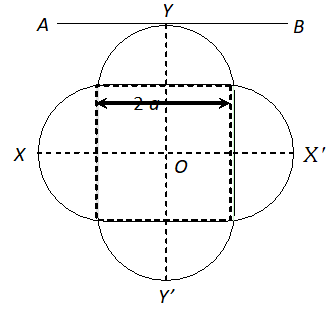since the lamina is symmetrical about the axes and $I_{x}=I_{y}$ $\therefore \quad I_{x}+I_{x}=I$ or $\quad I_{x}=\frac{1}{2} I=\frac{1}{2} \times 1.6 \mathrm{Ma}^{2}=0.8 \mathrm{Ma}^{2}$ According to the theorem of parallel axes, we have $I_{A B}=I_{x}+M(O Y)^{2}=0.8 M a^{2}+M(2 a)^{2}=4.8 \mathrm{Ma}^{2}$
Q. A ring, a disc and a sphere all of the same radius and same mass roll down an inclined plane from the same height h. Which of the three reaches the bottom (i) earliest (ii) latest ? [NCERT]
Ans. We know that acceleration of an object down an inclined plane is given by $a=\frac{g \sin \theta}{1+I / m r^{2}}$ ... (i) For a ring, $I=m r^{2}$ $a_{\sin g}=\frac{g \sin \theta}{1+1}=0.5 g \sin \theta$ For a disc, $I=\frac{1}{2} m r^{2}$ $\therefore \quad \frac{g \sin \theta}{1+\frac{1}{2}}=\frac{2}{3} g \sin \theta \quad=0.67 g \sin \theta$ For a sphere, $I=\frac{2}{5} m r^{2}$ $\therefore \quad a_{\text {sphere }}=\frac{g \sin \theta}{1+\frac{2}{5}}=\frac{5}{7} g \sin \theta=0.71 g \sin \theta$ As is maximum it will reach the bottom at the earliest. Again, as is minimum, it will reach the bottom at the end. The result does not depend upon the mass or the radices of the rolling body.
Q. A uniform bar of length 6a and mass 8m lies on a smooth horizontal surface. Two point masses m and 2m moving in the same plane with speed 2v and v respectively strike at a and 2a and stick to the rod. Find (i) Moment of Inertia of the system after the collision. (ii) Angular momentum associated (iii) Angular velocity, just after the collision
Ans. Angular momentum with the two masses before collision $L_{i}=m 2 v \cdot a+2 a 2 m v=6 m v a$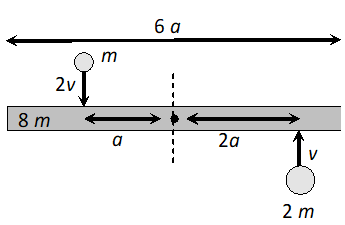moment of the bar about an axis through its central axis, $I_{b}=\frac{8 m(6 a)^{2}}{12}=\frac{8 \times 36 \times m a^{2}}{12}=24 m a^{2}$ (i) Moment of inertia after collision $=24 m a^{2}+m a^{2}+2 m \cdot 4 a^{2}$ $I_{f}=33 m a^{2}$ (ii) Angular momentum associated $=L_{i}=6 \mathrm{mva}$ (iii) Angular velocity after collision $=\omega=\frac{L_{i}}{I_{f}}$ i.e., $\quad \omega=\frac{6 m v a}{33 m a^{2}}=\frac{6 v}{33 a}=\frac{2 v}{11 a}$
Q. What will be the duration of the day, if the earth suddenly shrinks to $\frac{1}{64}$ of its original volume, mass remaining unchanged ?
Ans. Let $T_{1}$ and $T_{2}$ be the periods of revolution of theearth before and after its contraction ; and $R_{1}$ and $R_{2}$ be its radii before and after the contraction. Now, according to the principle of conservation of angular momentum, $I_{1} \omega_{1}=I_{2} \omega_{2}$ where $I_{1}=\frac{2}{5} M R_{1}^{2}$ and $I_{2}=\frac{2}{5} M R_{2}^{2}$ are moments of inertia of the earth before and after its contraction. it may be noted that the mass of the earth $(M)$ remains unchanged. $\therefore \frac{2}{5} M R_{1}^{2}\left(\frac{2 \pi}{T_{1}}\right)=\frac{2}{5} M R_{2}^{2}\left(\frac{2 \pi}{T_{2}}\right)$ $\Rightarrow T_{2}=\left(\frac{R_{2}}{R_{1}}\right)^{2} \times T_{1}$ ...(i) As the earth shrinks to $\frac{1}{64}$ of its volume, we have final volume $=\frac{1}{64}$ (initial volume) substituting the value of $\frac{R_{2}}{R_{1}}$ in equation (i) we get $T_{2}=\left(\frac{1}{4}\right)^{2} T_{1}=\frac{T_{1}}{16}$ i.e. duration of the day will be of 1.5 hour.
Q. Two discs of moments of inertia $\mathrm{I}_{1}$ and $\mathrm{I}_{2}$ and about their respective axis (normal to the disc and passing through the centre), and angular speed $\omega_{1}$ and $\omega_{2}$ and are brought into contact face to face with their axes of rotation coincident. (a) What is the angular speed of the two-disc system ? (b) Show that the kinetic energy of the combined system is less than the sum of the initial kinetic energies of the two discs. How do you account for this loss in energy ? Take $\omega_{1}^{1} \omega_{2}$.
Ans. (a) Total initial angular momentum of the two discs $=I_{1} \omega_{1}+I_{2} \omega_{2}$ since the two discs are brought into contact face to face, with their axes of rotation coincident, the M.I. of the combined system will be $I_{1}+I_{2} .$ If $\omega$ is the angular speed of the combined system, then final angular momentum of the system $=\left(I_{1}+I_{2}\right) \omega$ since no external torque acts on the system, we have $I_{1} \omega_{1}+I_{2} \omega_{2}=\left(I_{1}+I_{2}\right) \omega \Rightarrow \omega=\frac{I_{1} \omega_{1}+I_{2} \omega_{2}}{I_{1}+I_{2}}$ (b) Initial K.E. of rotation of the two discs $=\frac{1}{2} I_{1} \omega_{1}^{2}+\frac{1}{2} I_{2} \omega_{2}^{2}$ Final K.E. of the combined system $=\frac{1}{2}\left(I_{1}+I_{2}\right) \omega^{2}=\frac{1}{2}\left(I_{1}+I_{2}\right)\left(\frac{I_{1} \omega_{1}+I_{2} \omega_{2}}{I_{1}+I_{2}}\right)^{2}=\frac{1}{2} \frac{\left(I_{1} \omega_{1}+I_{2} \omega_{2}\right)^{2}}{I_{1}+I_{2}}$ Now, (initial K.E.) – (final K.E.) $=\frac{1}{2} I_{1} \omega_{1}^{2}+\frac{1}{2} I_{2} \omega_{2}^{2}-\frac{1}{2} \frac{\left(I_{1} \omega_{1}+I_{2} \omega_{2}\right)^{2}}{I_{1}+I_{2}}$ $=\frac{1}{2} \times \frac{I_{1}^{2} \omega_{1}^{2}+I_{1} I_{2} \omega_{1}^{2}+I_{1} I_{2} \omega_{2}^{2}+I_{2}^{2} \omega_{2}^{2}-I_{1}^{2} \omega_{1}^{2}-I_{2}^{2} \omega_{2}^{2}-2 I_{1} I_{2} \omega_{1} \omega_{2}}{I_{1}+I_{2}}$ $=\frac{I_{1} I_{2}}{2\left(I_{1}+I_{2}\right)}\left(\omega_{1}^{2}+\omega_{2}^{2}-2 \omega_{1} \omega_{2}\right)=\frac{I_{1} I_{2}\left(\omega_{1}-\omega_{2}\right)^{2}}{2 I_{1}+I_{2}}$ = a positive quantity Hence the rotational kinetic energy of the combined system is less than the sum of the initial energies of the two discs. The loss of energy is due to dissipation of energy in frictional contact of the two discs. It may be pointed out that the two discs rotate as a single combined system with a common angular speed, because of frictional contact only. However, the angular momentum will be conserved as the frictional torque is an internal torque.
eSaral provides you complete edge to prepare for Board and Competitive Exams like JEE, NEET, BITSAT, etc. We have transformed classroom in such a way that a student can study anytime anywhere. With the help of AI we have made the learning Personalized, adaptive and accessible for each and every one. Visit eSaral Website to download or view free study material for JEE & NEET. Also get to know about the strategies to Crack Exam in limited time period.